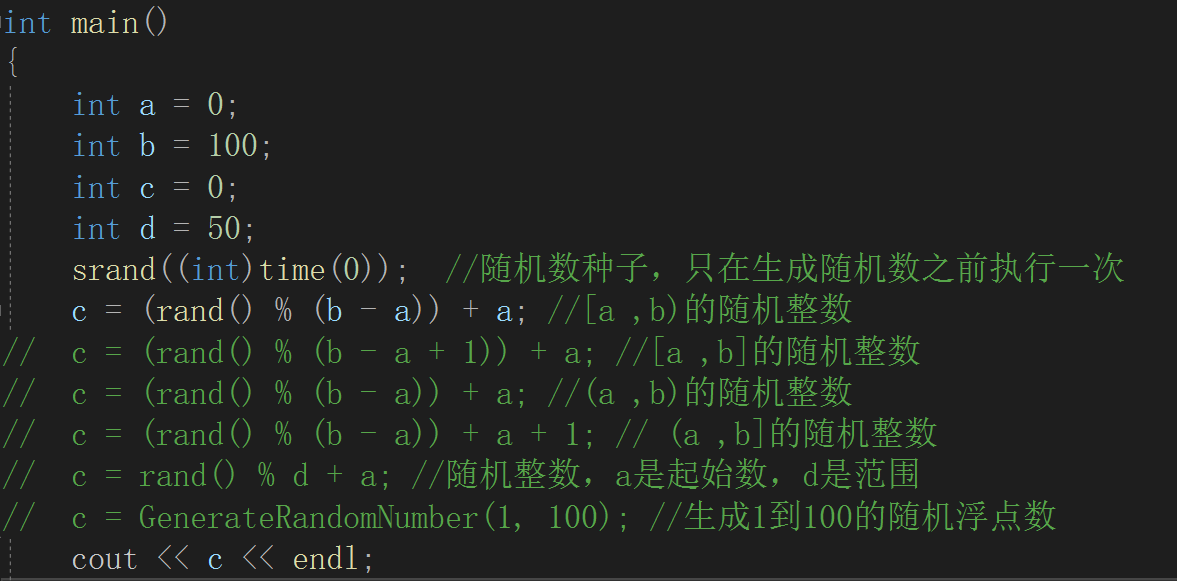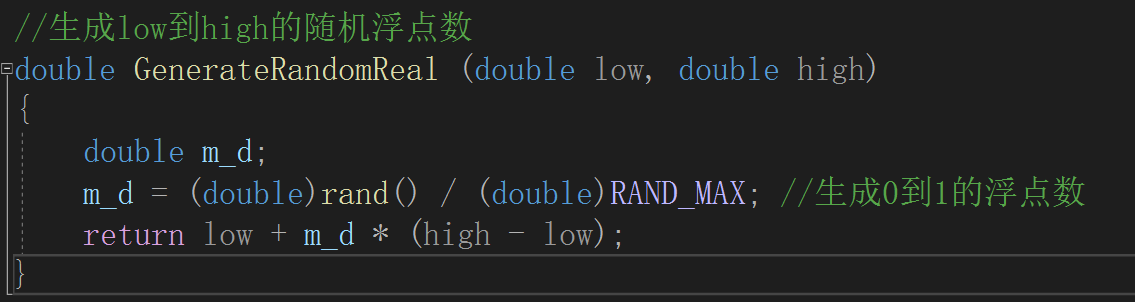• C++生成随机数（压缩包里包含源文件和已经编译好的exe文件）
• c++生成随机数 随机浮点数的函数 代码 main.cpp #include <iostream> #include <time.h> #include <stdlib.h> #include "random.h" using namespace std; int main() { int a = 0; int b = ...
c++生成随机数随机浮点数的函数代码
main.cpp
#include <iostream>
#include <time.h>
#include <stdlib.h>
#include "random.h"

using namespace std;

int main()
{
int a = 0;
int b = 100;
int c = 0;
int d = 50;
srand((int)time(0));  //随机数种子，只在生成随机数之前执行一次
c = (rand() % (b - a)) + a; //[a ,b)的随机整数
//	c = (rand() % (b - a + 1)) + a; //[a ,b]的随机整数
//	c = (rand() % (b - a)) + a; //(a ,b)的随机整数
//	c = (rand() % (b - a)) + a + 1; // (a ,b]的随机整数
//	c = rand() % d + a; //随机整数，a是起始数，d是范围
//	c = GenerateRandomNumber(1, 100); //生成1到100的随机浮点数
cout << c << endl;

return 0;
}

random.h
double GenerateRandomReal(double low, double high);


random.cpp
#include <iostream>
#include <time.h>
#include <stdlib.h>

using namespace std;

//生成low到high的随机浮点数
double GenerateRandomReal (double low, double high)
{
double m_d;
m_d = (double)rand() / (double)RAND_MAX; //生成0到1的浮点数
return low + m_d * (high - low);
}


接下来是我写的随机数生成库，供大家参考
源代码
main.cpp
#include <iostream>
using namespace std;
#include "random.h"

int main()
{
int i;
Randomize();
for (i = 0; i <= 10; i++)
{
int t = GenerateRandomNumber(1, 100);
cout << t << "  ";
}
cout << endl;

for (i = 0; i <= 10; i++)
{
double d = GenerateRandomReal(1, 100);
cout << d << "  ";
}
cout << endl;
return 0;
}

random.h
void Randomize();
int GenerateRandomNumber(int low,int high);
double GenerateRandomReal(double low,double high);

random.cpp
#include <iostream>
#include <cstdlib>
#include <ctime>

using namespace std;

void Randomize()
{
srand((int)time(0));
}

int GenerateRandomNumber(int low, int high)
{
double m_d;
if (low > high)
{
cout << "GenerateRandomNumber:error,make sure low <= high" << endl;
exit(1);
}
m_d = rand() / ((double)RAND_MAX + 1.0);

return low + (int)(m_d * ((double)high - (double)low + 1.0));

}

double GenerateRandomReal(double low, double high)
{
double m_d;
if (low > high)
{
cout << "GenerateRandomReal:error,make sure low <= high" << endl;
exit(1);
}
m_d = (double)rand() / (double)RAND_MAX;

return low + m_d * (high - low);

}

这就是c++生成随机数的实现了，如有错误，欢迎指正


展开全文• c++ 生成随机数 #include <iostream> #include <ctime> #include <cstdlib> using namespace std; int main() { int i,j; srand( (unsigned)tim...


c++ 生成随机数

#include <iostream>
#include <ctime>
#include <cstdlib>

using namespace std;

int main()

{
int i,j;
srand( (unsigned)time(NULL));

/*生成10个随机数*/

for (i=0;i<10;i++)
{
//生成实际随机数
j=rand();

cout << "随机数：　"<<j <<endl;

}
return 0;
}


随机数：　1563984234
随机数：　1493872638
随机数：　742794530
随机数：　846192927
随机数：　601279458
随机数：　113844087
随机数：　839863344
随机数：　1536651017
随机数：　575020234
随机数：　373566792


posted on 2019-03-13 11:57 luoganttcc 阅读(...) 评论(...)  编辑 收藏


展开全文• c++写了个蚁群算法，发现用到c++随机数这里还是问题多多，下面就来总结一下： 首先调用rand()函数可以生成一个0-RAND_MAX之间的随机数，RAND_MAX的值为32767； 然后假设我输出一个rand()之后，重新编译运行代码...

用c++写了个蚁群算法，发现用到c++随机数这里还是问题多多，下面就来总结一下：
首先调用rand()函数可以生成一个0-RAND_MAX之间的随机数，RAND_MAX的值为32767；
然后假设我输出一个rand()之后，重新编译运行代码，这个值不会变，
想要得到一个新的随机数，这里就要用到随机数种子初始化函数srand()
一般都是选择系统时间作为种子
即srand((unsigned int)time(NULL))
下面上个简单的代码

#include<stdio.h>
#include<stdlib.h>
#include<time.h>
#include<conio.h>
#include<iostream>
#define N 10
#define M 1010
using namespace std;
int main()
{
double num,i;
srand((unsigned int)time(NULL));//产生随机数的种子
cout<<RAND_MAX<<endl;
for(i=1;i<=N;i++)
{
num= (double)(rand()%10000)/10000;//产生随机数
cout<<num<<endl;
}

}


注意千万不能把srand函数放到循环体里面，如下：

for(i=1;i<=N;i++)
{
srand((unsigned int)time(NULL));//产生随机数的种子
num= (double)(rand()%10000)/10000;//产生随机数
cout<<num<<endl;
}


这样会输出N个相同的值（当N不是很大的时候），因为做N次循环的时间太短了，所以相当于给这N个数都初始化了相同的种子
最后就是如何生成大于RAND_MAX的随机数的问题
似乎不能直接修改宏定义，所以目前还没有想到解决的方法

转载于:https://www.cnblogs.com/EdwardZhang/p/10779825.html
展开全文• 主要介绍了C++生成随机数的实现代码，文中通过示例代码介绍的非常详细，对大家的学习或者工作具有一定的参考学习价值，需要的朋友们下面随着小编来一起学习学习吧...

# c++生成随机数c++ 订阅